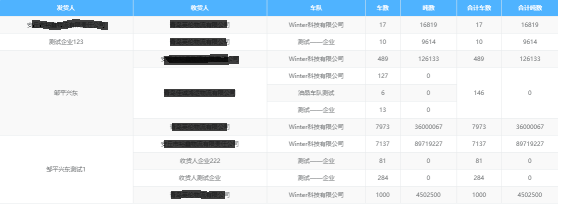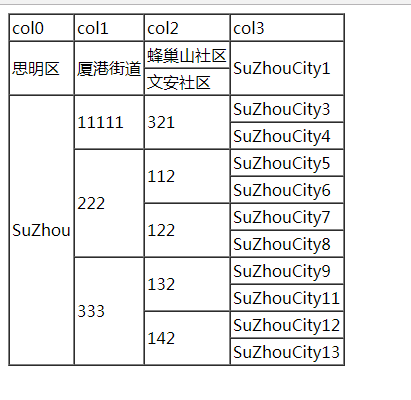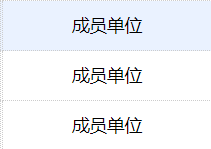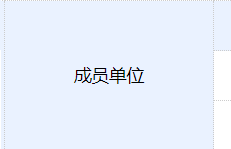• 通过js合并表格相邻的重复的单元格
js必须等表格加载完成后调用。
效果如图：js代码：

function uniteTable(tableId,colLength) {
//colLength-- 需要合并单元格的列1开始
var tb=document.getElementById(tableId);

tb.style.display='';

var i = 0;
var j = 0;

var rowCount = tb.rows.length; //   行数
var colCount = tb.rows.cells.length; //   列数

var obj1 = null;
var obj2 = null;
var obj3 = null;

//为每个单元格命名
for (i = 0; i < rowCount; i++) {
for (j = 0; j < colCount; j++) {
tb.rows[i].cells[j].id = "tb__" + i.toString() + "_" + j.toString();
}
}

//合并行
for (i = 0; i < colCount; i++) {
if (i == colLength) break;
obj1 = document.getElementById("tb__0_" + i.toString())
for (j = 1; j < rowCount; j++) {
obj2 = document.getElementById("tb__" + j.toString() + "_" + i.toString());
if (obj1.innerText == obj2.innerText) {
obj1.rowSpan++;
obj2.parentNode.removeChild(obj2);
} else {
obj1 = document.getElementById("tb__" + j.toString() + "_" + i.toString());
}
}
}

//合并列
for (i = 0; i < rowCount; i++) {
colCount = tb.rows[i].cells.length;
obj1 = document.getElementById(tb.rows[i].cells.id);
for (j = 1; j < colCount; j++) {
if (j >= colLength) break;
if (obj1.colSpan >= colLength) break;
obj2 = document.getElementById(tb.rows[i].cells[j].id);
if (obj1.innerText == obj2.innerText) {
obj1.colSpan++;
obj2.parentNode.removeChild(obj2);
j = j - 1;
}
else {
obj1 = obj2;
j = j + obj1.rowSpan;
}
}
}
}

优化后的效果（这里只实现了合并行）：
效果图如下：代码如下：

function uniteTable(tableId,colLength) {
//colLength-- 需要合并单元格的列1开始
var tb=document.getElementById(tableId);

tb.style.display='';

var i = 0;
var j = 0;

var rowCount = tb.rows.length; //   行数
var colCount = tb.rows.cells.length; //   列数

var obj1 = null;
var obj2 = null;
var obj3 = null;

//为每个单元格命名
for (i = 0; i < rowCount; i++) {
for (j = 0; j < colCount; j++) {
tb.rows[i].cells[j].id = "tb__" + i.toString() + "_" + j.toString();
}
}

//合并行  （列循环）第一行第一列均从0开始
for (i = 0; i < colCount; i++) {

//如果第3，4，5列不进行合并操作
if (i == 2 || i == 3 || i == 4) continue;
obj1 = document.getElementById("tb__0_" + i.toString())
//（行循环）
for (j = 1; j < rowCount; j++) {
obj2 = document.getElementById("tb__" + j.toString() + "_" + i.toString());

if(i == 0){         //第1列全部进行合并操作
if (obj1.innerText == obj2.innerText) {     //判断值是否相等
obj1.rowSpan++;                         //合并行
obj2.parentNode.removeChild(obj2);      //移除被合并的行
} else {
obj1 = document.getElementById("tb__" + j.toString() + "_" + i.toString());
}
}else if(i == 5){   //第6列合并操作参照第2列，第二列合并了，第5列才进行合并
obj3 = document.getElementById("tb__" + j.toString() + "_" + (i-4).toString());
if (obj1.innerText == obj2.innerText && obj3 == null) {
obj1.rowSpan++;
obj2.parentNode.removeChild(obj2);
} else {
obj1 = document.getElementById("tb__" + j.toString() + "_" + i.toString());
}
}else{              //只有前一列进行了合并操作后面的才会进行合并操作
obj3 = document.getElementById("tb__" + j.toString() + "_" + (i-1).toString());
if (obj1.innerText == obj2.innerText && obj3 == null) {
obj1.rowSpan++;
obj2.parentNode.removeChild(obj2);
} else {
obj1 = document.getElementById("tb__" + j.toString() + "_" + i.toString());
}
}
}
}
}
展开全文javascript
• 这几天做一个项目有用到表格显示数据的地方，客户要求重复的数据列需要合并，就总结了一下GridView 和 Repeater 关于重复数据合并的方法。 效果图如下 ： GridView ： 前台代码 ： 一级> <asp:...
• 这几天做一个项目有用到表格显示数据的地方，客户要求重复的数据列需要合并，就总结了一下GridView 和 Repeater 关于重复数据合并的方法。 效果图如下： GridView ： 前台代码 ： 一级> <asp:Label ID=...
• // 我们对每一行数据进行"扫面"处理 关键是定位col-td, 和其对应的rowspan $('tbody tr',$table).each(function (index) { // td:eq的colIndex即列索引 var $td =$('td:eq(' + colIndex + ')', this); //...<!DOCTYPE html>
<html xmlns="http://www.w3.org/1999/xhtml">

<meta http-equiv="Content-Type" content="text/html; charset=utf-8" />
<title></title>
<script src="jquery-1.11.1.min.js"></script>

<body>
<tr>
<td>col0</td>
<td>col1</td>
<td>col2</td>
<td>col3</td>
</tr>
<tbody>
<tr>
<td>思明区</td>
<td>厦港街道</td>
<td> 蜂巢山社区</td>
<td>SuZhouCity1</td>
</tr>
<tr>
<td>思明区</td>
<td>厦港街道</td>
<td> 文安社区</td>
<td>SuZhouCity1</td>
</tr>
<tr>
<td>SuZhou</td>
<td>11111</td>
<td>321</td>
<td>SuZhouCity3</td>
</tr>
<tr>
<td>SuZhou</td>
<td>11111</td>
<td>321</td>
<td>SuZhouCity4</td>
</tr>
<tr>
<td>SuZhou</td>
<td>222</td>
<td>112</td>
<td>SuZhouCity5</td>
</tr>
<tr>
<td>SuZhou</td>
<td>222</td>
<td>112</td>
<td>SuZhouCity6</td>
</tr>
<tr>
<td>SuZhou</td>
<td>222</td>
<td>122</td>
<td>SuZhouCity7</td>
</tr>
<tr>
<td>SuZhou</td>
<td>222</td>
<td>122</td>
<td>SuZhouCity8</td>
</tr>
<tr>
<td>SuZhou</td>
<td>333</td>
<td>132</td>
<td>SuZhouCity9</td>
</tr>
<tr>
<td>SuZhou</td>
<td>333</td>
<td>132</td>
<td>SuZhouCity11</td>
</tr>
<tr>
<td>SuZhou</td>
<td>333</td>
<td>142</td>
<td>SuZhouCity12</td>
</tr>
<tr>
<td>SuZhou</td>
<td>333</td>
<td>142</td>
<td>SuZhouCity13</td>
</tr>

</tbody>
</table>
<script>
$(function () { // 看过jquery源码就可以发现$.fn就是$.prototype, 只是为了兼容早期版本的插件 // 才保留了jQuery.prototype这个形式$.fn.mergeCell = function (options) {
return this.each(function () {
var cols = options.cols;
for (var i = cols.length - 1; cols[i] != undefined; i--) {
// fixbug console调试
// console.debug(cols[i]);
mergeCell($(this), cols[i]); } dispose($(this));
});
};

function mergeCell($table, colIndex) {$table.data('col-content', ''); // 存放单元格内容
$table.data('col-rowspan', 1); // 存放计算的rowspan值 默认为1$table.data('col-td', $()); // 存放发现的第一个与前一行比较结果不同td(jQuery封装过的), 默认一个"空"的jquery对象$table.data('trNum', $('tbody tr',$table).length); // 要处理表格的总行数, 用于最后一行做特殊处理时进行判断之用
// 我们对每一行数据进行"扫面"处理 关键是定位col-td, 和其对应的rowspan
$('tbody tr',$table).each(function (index) {
// td:eq中的colIndex即列索引
var $td =$('td:eq(' + colIndex + ')', this);
// 取出单元格的当前内容
var currentContent = $td.html(); // 第一次时走此分支 if ($table.data('col-content') == '') {
$table.data('col-content', currentContent);$table.data('col-td', $td); } else { // 上一行与当前行内容相同 if ($table.data('col-content') == currentContent) {
// 上一行与当前行内容相同则col-rowspan累加, 保存新值
var rowspan = $table.data('col-rowspan') + 1;$table.data('col-rowspan', rowspan);
// 值得注意的是 如果用了$td.remove()就会对其他列的处理造成影响$td.hide();
// 最后一行的情况比较特殊一点
// 比如最后2行 td中的内容是一样的, 那么到最后一行就应该把此时的col-td里保存的td设置rowspan
if (++index == $table.data('trNum'))$table.data('col-td').attr('rowspan', $table.data('col-rowspan')); } else { // 上一行与当前行内容不同 // col-rowspan默认为1, 如果统计出的col-rowspan没有变化, 不处理 if ($table.data('col-rowspan') != 1) {
$table.data('col-td').attr('rowspan',$table.data('col-rowspan'));
}
// 保存第一次出现不同内容的td, 和其内容, 重置col-rowspan
$table.data('col-td',$td);
$table.data('col-content',$td.html());
$table.data('col-rowspan', 1); } } }); } // 同样是个private函数 清理内存之用 function dispose($table) {
$table.removeData(); }$('#process').mergeCell({
// 目前只有cols这么一个配置项, 用数组表示列的索引,从0开始
// 然后根据指定列来处理(合并)相同内容单元格
cols: [0, 1, 2, 3]
});
});
//; (function ($) { //})(jQuery); function dd() { // 这里写成了一个jquery插件的形式$('#process').mergeCell({
// 目前只有cols这么一个配置项, 用数组表示列的索引,从0开始
// 然后根据指定列来处理(合并)相同内容单元格
cols: [0, 1, 2, 3]
});
}
</script>
</body>

</html>


展开全文• 首先合并单元格，既然是合并，肯定是要把相同的数据用一个单元格来完成。就是把这样的格式合并成这样的边框比较浅，图片可能不太清楚，但是能看得出来左边是三个单元格，右边是合并成了一个。要想实现这样的效...
前些天接到组长分配的任务，让我这个小菜鸟实现单元格的合并。刚开始觉得很复杂，可是仔细思考过后其实还是很简单的，下面就和大家分享一下自己每次的方法和存在的缺点以及如何修改和最终的成品，希望能够对大家有些帮助。首先合并单元格，既然是合并，肯定是要把相同的数据用一个单元格来完成。就是把这样的格式合并成这样的边框比较浅，图片可能不太清楚，但是能看得出来左边是三个单元格，右边是合并成了一个。要想实现这样的效果就要有一个前提，就是相同数据应该在一起。所以后台不能直接将数据库的数据list出来（数据库数据是没有顺序的，相同数据也没有在一起）。所以我们在select *的时候需要先对数据进行排序或者分组，然后再传到前台，排序时候直接order by 字段一，字段二，字段三.... 这里面排序多个字段的含义就是在字段一相等的前提下再进行字段二的排序。所以很显然，前台要合并的数据顺序是字段一>字段二>字段三>....。将数据排序好传到前台以后，前台就要进行判断了，当数据一样时进行单元格的合并。起初合并一列单元格的时候方法如下：function hebing1(col){
var trs = $("table tr"); var rows = 1; for(var i=trs.length;i>0;i--){ var cur =$($(trs[i]).find("td")[col]).text(); var next =$($(trs[i-1]).find("td")[col]).text(); if(cur==next){ rows++;$($(trs[i]).find("td")[col]).remove(); } else {$($(trs[i]).find("td")[col]).attr("rowspan",rows); rows=1; } } }这样很容易就实现了。for循环是根据你每页多少条数据，从下往上进行判断然后合并。如果从上往下合并，将代码改为for(var i=1;i<trs.length;i++){}之后前台就不能实现了。具体原因不清楚，应该是和下面的remove（）和rowspan方法有关，奈何自己js学的太烂，并没有研究透。可能当合并多个列的时候就会出现新的问题，因为你在合并一个单元格的时候要对左边的单元格进行判断，当左边单元格数据一样的时候进行合并，不一样的时候即使右边单元格数据一样也不能进行合并。这样实现也不难，只需要多获取两个单元格进行判断就可以了。同样合并三列的时候无非又多获取两个单元格进行判断。代码如下： function hebing2(col){ var trs =$("table tr");
var rows = 1;
for(var i=trs.length;i>0;i--){
var cur = $($(trs[i]).find("td")[col]).text();
var next = $($(trs[i-1]).find("td")[col]).text();
var parentId1 = $($(trs[i]).find("td")).text();
var parentId2 = $($(trs[i-1]).find("td")).text();
if(cur == next&&parentId1 == parentId2){
rows++;
$($(trs[i]).find("td")[col]).remove();
} else {
$($(trs[i]).find("td")[col]).attr("rowspan",rows);
rows=1;
}
}
}
function hebing3(col){
var trs = $("table tr"); var rows = 1; for(var i=trs.length;i>0;i--){ var cur =$($(trs[i]).find("td")[col]).text(); var next =$($(trs[i-1]).find("td")[col]).text(); var parentId1 =$($(trs[i]).find("td")).text(); var parentId2 =$($(trs[i-1]).find("td")).text(); var company1 =$($(trs[i]).find("td")).text(); var company2 =$($(trs[i-1]).find("td")).text(); if(cur == next&&parentId1 == parentId2&&company1 == company2){ rows++;$($(trs[i]).find("td")[col]).remove(); } else {$($(trs[i]).find("td")[col]).attr("rowspan",rows); rows=1; } } } function hebing4(col){ var trs =$("table tr");
var rows = 1;
for(var i=trs.length;i>0;i--){
var cur = $($(trs[i]).find("td")[col]).text();
var next = $($(trs[i-1]).find("td")[col]).text();
var parentId1 = $($(trs[i]).find("td")).text();
var parentId2 = $($(trs[i-1]).find("td")).text();
var company1 = $($(trs[i]).find("td")).text();
var company2 = $($(trs[i-1]).find("td")).text();
var position1 = $($(trs[i]).find("td")).text();
var position2 = $($(trs[i-1]).find("td")).text();
if(cur == next&&parentId1 == parentId2&&company1 == company2&&position1 == position2){
rows++;
$($(trs[i]).find("td")[col]).remove();
} else {
$($(trs[i]).find("td")[col]).attr("rowspan",rows);
rows=1;
}
}
}方法名里面的234就是要合并的是第几列，以hebing4（）这个方法为例，不难发现我们在进行判断的时候要判断123三列的数据是否相同，只有数据都相同了才进行合并。然后前台初始化完成后只需要调用这几个方法就行了。例如：hebing4（4）；  hebing3（3）；  hebing2（2）；   hebing1（1）；这个括号里面的参数和方法名里面的数字意义是一样的，表示要合并的是第几列，不能省略。有人可能发现了这里是从第四列开始合并的，然后是第三列，第二列，第一列。而不是从第一列开始。这是因为如果先合并了第一列，把第一列某些单元格内容清空了，再合并后面的列的时候要获取前面列的数据进行判断，这时候就会出错误。所以要切记从右往左合并。但是我们又发现了，这样代码很多且大部分都是重复的，而且多一列就要多写一个方法，很麻烦，于是我想到应该用嵌套循环的方法将几个方法放在一起。最后得到了下列这段代码：      //合并单元格,参数为合并的列数
function hebing(col){
var trs = $("table tr"); var rows = 1; for(var j=col;j>0;j--){ for(var i=trs.length;i>0;i--){ for(var k=j;k>0;k--){ var cur =$($(trs[i]).find("td")[k]).text(); var next =$($(trs[i-1]).find("td")[k]).text(); if(cur!=next){$($(trs[i]).find("td")[j]).attr("rowspan",rows); rows=1; break; }else if(k==1){ rows++;$($(trs[i]).find("td")[j]).remove(); } } } } }这里的参数是要合并的列数，从第一列算起，中间不能间断，不能合并1和3两列。这里用到了三个循环。最外面的循环是根据列数判断要合并多少列，中间的循环是根据数据的条数进行相应次数的判断。最重要的是最里面的循环，这个循环是要看该列前面有多少列，进行多少次判断。注意K=J，J=col,但是不能写成K=col。而且什么时候用K什么时候用J一定要很清楚。使用时候直接调用这一个方法就好了，比之前的方便很多。实现的效果由于项目要保密的关系就不贴出来给大家看了。希望这个方法对大家有帮助。说到这里可能又会有新的问题，比如如果数据很多，在进行排序时候加载数据特别慢怎么办？这里我也在思考这个问题，目前想到的解决方法是利用聚集索引直接改变数据库中数据的顺序，就不再需要order by了。关于聚集索引和非聚集索引我还分享过一个笔记，大家可以去看一下。http://blog.csdn.net/sun337939896/article/details/79218522最后说一下自己的感悟：通过这一个小小的功能的实现反映出我们进行项目开发的过程，从无到有，从复杂到简单，要一步一个脚印。如果你一直想着一开始就实现最终这个方法，而忽略其中的过程，那么我相信你很难做到。如果大家急着用可以直接拿去最后的代码。如果大家和我一样处在学习成长阶段，建议从最开始的一列到多列，从多个方法到一个方法一步一步来，这个过程远比最后的结果重要很多。注：本人尚未毕业，才疏学浅，目前能想到的方法就是这个，肯定还有很多更好的方法，大家也可以拿出来共勉。不喜勿喷。 展开全文排序 • 在Access创建对Excel工作簿的链接：在Access选择“外部数据——导入——Excel”，在“获取外部数据”对话框选择“通过创建链接表来链接到数据源”。咱们假设链接表为sheet1(合并表1)、sheet2(合并表2)、sheet3... office 2007的操作方法： 1.在Access中创建对Excel工作簿的链接：在Access中选择“外部数据——导入——Excel”，在“获取外部数据”对话框中选择“通过创建链接表来链接到数据源”。咱们假设链接表为sheet1(合并表1)、sheet2(合并表2)、sheet3(合并后数据存放)。 注意：必须为每个Excel工作簿重复该过程直到将它们都链接到数据库。 2.在SQL视图中开始新的查询，单击“创建——其他——查询设计”。选择“查询工具-设计”中的“SQL视图”。 3.在SQL视图中，为所有链接的Excel表输入Select语句，实现语句：insert into sheet3 select * from(select * from sheet1 union select * from sheet2) t，直接拷过去即可，然后点击执行。 相关解释：默认情况下，UNION操作符会移除数据中的任何重复行。如果知道数据中有重复行，并且想保留它们，那么需要使用UNION ALL，以允许传递重复行。 展开全文excel access sql insert • 现在有了这款【多个Excel合并重复数据过滤器】软件，你就可以轻松去除重复数据了。 1）你可以导入多个，甚至是大量EXCEL文档，几个、几十个、几百个... 2）导入数据后，你可以选择对某列数据进行过滤，去除重复... • 合并表格的【第一列】相同数据： //需要自己引入jq$(function() { \$("#table").rowspan(0); //传入的参数是对应的列数从0开始 第一列合并相同 //如果想后面的列也合并，就0,1,2,3依次往后推算 }); jQuery.fn....
• 相邻表格相同数据看起来不好看，而且显得冗余，如果将相邻表格的相同数据进行合并处理，不仅看起来美观很多，而且显得更加的专业。
• c#中合并DataTable重复行的值，需要的朋友可以参考一下
• python excel 多个文件合并 按一定规则去重 生成一张新的表 xlrd xlwt
• 即为重复值，图中用红色标识，现在的需求就是从两列中提取唯一值到其他区域，多次出现的重复值只提取一次，下面以Excel 2013和下图两列数据为例向大家介绍使用四种不同的方法从两列数据中提取不重复值，Excel
• ///合并table某行列重复值 tableid:table的id;stardcolumnindex:参考列的内容进行对比;controlcolumnindex控制合并的列 function MerginCells(tableid, stardcolumnindex, controlcolumnindex, ishidden) { var...Jquery
• 从数据库或PB中转的数据到Excel，为了便于阅读，通常希望把某些列的上下行相同的数据合并单元格，查看Excel好像没有此功能，于是通过PB来完成了该需求。 如下代码： String docname,named String current_...excel
• 数据整体（不含待求和数据）复制到与原数据同一表格但不同行列； 如下图所示 在新表格填充数据的位置输入公式：SUMIF(条件区域，条件，求和数据区域)； 条件区域：原表格所有数据；条件：删除重复项时所...
• 基于vue 通过数据过滤 实现表格合并 需求 基于vue渲染的数据表格 需要对相同的列进行合并 思路 自从使用了vue，就再也不想直接操作dom节点了，所以还是对数据操作。 要合并单元格需要用到rowspan属性，...vue
• 可以看到此数据中重复姓名 然后复制B列数据粘贴到E列中 点击数据选择删除重复项 选中“以当前选定区域排序”点击删除重复项 选择数据包含标题,再点击确定。 弹出删除重复项对话框，点击确定E列就得到不重复的...
• R语言筛选V2和V3两列，元素的重复次数超过2次以上的数据，其中2和3的重复次数超过3次，需要筛选出来。筛选不同门店的数量，进行数据计数和统计。
• 数据的拆分合并提取 删除错误 转置与反转 透视和逆透视（二维表与一维表的转换） 数据格式的转换 如下图，我们有一组数据，但是发现其格式很不符合我们建模数据的需求，需要进行处理（比如姓名列有回车、空格...
• （1）查找硬盘的所有重复文件，并列出每组重复文件每个文件的文件名、文件大小、位置和创建日期【不是重名文件!!用md5值判断是否是相同文件】 import hashlib import os from collections import Counter import...
• 找了很多资料想要将自己数据集中相同id的行进行整合，（单纯数据合并）： 例如数据： id name review 1 mi good 1 mi great 2 k nice 2 k really 合并之后效果： id name review 1 mi [good,great] 2 k [nicepython
• 本软件能实现两个excel文件之间整条数据按某一个列进行强制匹配（excel表格自带匹配功能无法匹配时，可使用本软件解决），并将匹配成功的行数据合并为一整行，之后输出到新的excel。（匹配的源文件，未匹配成功...
• 页面的table表格中又是需要将相同的数据合并，今天就学到了一个简单实用有效的方法： Document 111 222 333 111 555 333合并 table
• [SQL]合并一个表里面的的多行数据(如果不重复合并，如果重复则舍弃) 今天我在CSDN里提的一個問題，非常感謝馬可老大~-------------------------------------------------------------------------测试数据编号 ...sql insert function table
• 数据合并:通过堆叠合并和主键合并等多种合并的方式可以将关联的数据信息合并在同一张表格中 数据清洗：对数据进行检测，查询是否有重复值，缺失值和异常值，并对这些数据进行处理 标准化数据：为了消除特征之间...
• Vue_Elenent 表格相同数据列进行合并 作者：秋名 思路：后台查询表格数据，查询出来后直接传到前端，前端通过foreach循环，然后对相同的表格进行合并。（同一个表格，但是每一行，固定一列的数据都相同，即可使用......